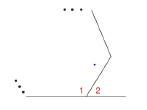Chapter 2.5, Problem 32E### Elementary Geometry for College St...

6th Edition
Daniel C. Alexander + 1 other
ISBN: 9781285195698

#### Solutions

Chapter
Section### Elementary Geometry for College St...

6th Edition
Daniel C. Alexander + 1 other
ISBN: 9781285195698
Textbook Problem
1 views

# The adjacent interior and exterior angles of a polygon are supplementary, as indicated in the drawing. Assume that you know that the measure of each interior angle of a regular polygon is ( n − 2 ) 180 ° n . a) Express the measure of each exterior angle as the supplement of the interior angle.b) Simplify the expression in part (a) to show that each exterior angle has a measure of 360 n.

To determine

a)

To find:

The measure of each exterior angle as supplement of the interior angle.

Explanation

Given:

The following figure shows the given diagram.

The measure of each interior angle of a regular polygon is (n2)180°n

Approach:

(1). 2 is supplementary of 1.

(2). The sum of measure of two supplementary angles will be 180°.

Calculation:

The measure of each interior angle of a regular polygon is (n2)180°n.

m1=(n2)180°n

In the given figure, 2 is exterior angle to the adjacent interior angle 1

To determine

b)

To simplify:

The expression in part (a) to show that each exterior angle has a measure of 360n.

### Still sussing out bartleby?

Check out a sample textbook solution.

See a sample solution

#### The Solution to Your Study Problems

Bartleby provides explanations to thousands of textbook problems written by our experts, many with advanced degrees!

Get Started

#### In Exercises 49-62, find the indicated limit, if it exists. 55. limx1xx1

Applied Calculus for the Managerial, Life, and Social Sciences: A Brief Approach

#### Simplify the expressions in Exercises 97106. 21/a/22/a

Finite Mathematics and Applied Calculus (MindTap Course List)

#### True or False: A straight (simple) substitution may be used to evaluate 2+lnxxdx.

Study Guide for Stewart's Single Variable Calculus: Early Transcendentals, 8th